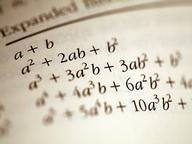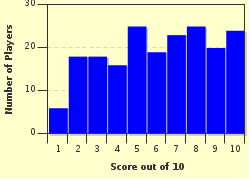FREE! Click here to Join FunTrivia. Thousands of games, quizzes, and lots more!# Enter the Matrix Trivia Quiz

### Matrices are often ignored in high school math but actually play a large part in college math. See how well you know your matrices?

A multiple-choice quiz by redsoxfan325. Estimated time: 5 mins.

Author
redsoxfan325
Time
5 mins
Type
Multiple Choice
Quiz #
293,960
Updated
Dec 13 22
# Qns
10
Difficulty
Tough
Avg Score
6 / 10
Plays
1092
1. How do you add matrices? Hint

Add the rows of one with the columns of another
Add all the numbers in both matrices together to get a number
Add the corresponding components of the matrices

#### NEXT>

2. How do you multiply matrices? Hint

Take the dot product of the rows of the first with the columns of the second
Take the dot product of the columns of the first with the rows of the second
Multiply corresponding components
You can't multiply matrices

#### NEXT>

3. How do you divide matrices? Hint

You divide the corresponding components in each matrix
Take the cross product of the rows of the first with the columns of the second
Take the cross product of the columns of the first with the rows of the second
You can't divide matrices

#### NEXT>

4. Row-reduction, also know as Gauss-Jordan Elimination, can be used for which of the following? Hint

All of these
Finding the determinant of the matrix
Solving systems of equations using an augmented matrix
Finding the rank of the matrix

#### NEXT>

5. Finding the inverse of a matrix involves augmenting the matrix by the identity matrix and performing row reduction until Hint

all the terms in the "body" part of the matrix are zero
the "body" part of the matrix looks like the identity matrix
the "body" part of matrix is the same as the augmented part

#### NEXT>

6. This matrix operation tells you whether or not the matrix is invertible. It is also useful for telling you area change during linear transformations, finding eigenvectors, and using Cramer's Rule to solve equations. Hint

discriminant
determinant
derivative
demonstrative

#### NEXT>

7. So now that you know the matrix operations, how about a few examples before we move on? Find the determinant of:

[3 -5] [-2 4]
[8 -1]+[-7 0]
Hint

3
1
0
2

#### NEXT>

8. Solve this system:
2x-4y+6z=10
y+ z=-2
x+2y+3z=-3
Hint

x=1, y=-2, z=0
x=-2, y=0, z=1
x=0, y=1, z=-2
This system is unsolvable.

#### NEXT>

9. For any given matrix A, any vector x such that Ax=0 is said to be in the ________ of A, and all linearly independent column vectors of A are said to make up the _________ of A. Hint

kernel; image
basis; space
image; kernel
space; basis

#### NEXT>

10. For any given 3x3 matrix the dimension of the kernel (number of vectors in the kernel) is the same as the dimension of the image (number of vectors in the image). Hint

always true
never true
sometimes true
not enough information

 (Optional) Create a Free FunTrivia ID to save the points you are about to earn:Select a User ID:Choose a Password:Your Email:

Most Recent Scores
Sep 24 2023 : mariappank511: 0/10
Sep 23 2023 : AdamM7: 8/10

Score DistributionQuiz Answer Key and Fun Facts
1. How do you add matrices?

Adding matrices is one of the simplest operations you can perform. Subtraction works the same way: subtract the corresponding components of each matrix.

Note: Matrices must have the same dimension in order for you to add/subtract them.
2. How do you multiply matrices?

Answer: Take the dot product of the rows of the first with the columns of the second

If you think of the first entry of the product matrix as entry 1,1 (row, column), the dot product of the 1st row of the first matrix with the 1st column of the second matrix will be entry 1,1. The dot product of the 1st row of the first matrix with the 2nd column of the second matrix will be entry 1,2. The dot product of the 2nd row of the first matrix with the 1st column of the second matrix will be entry 2,1 and so on until you have exhausted all row-column combinations.

Note: The matrices don't have to be the same dimension to multiply them. In order to multiply them, the number of columns in the first matrix has to be the same as the number of rows in the second matris. The dimension of the resulting product matrix will always have the same number of rows as the first matrix and the same number of columns as the second matrix.
3. How do you divide matrices?

You can't divide matrices. The way you would get around this is by multiplying by the inverse of the matrix. If you think about simple numbers, 12/4 is the same as 12*(1/4). Finding the inverse of a matrix will be discussed later in this quiz.
4. Row-reduction, also know as Gauss-Jordan Elimination, can be used for which of the following?

You can multiply rows by scalars and add them together to get new rows to replace the old ones with the goal of achieving the identity matrix. An augmented matrix is a matrix with more columns than rows. The most common augmented matrix is one where there is one more column than row, and you place the coefficients of the equations in the "body" of the matrix and the numbers the equations equal in the augmented part of the matrix (the last column). Once you have obtained the identity matrix in the "body", whatever is in the last column will be your solutions to the system.

Finding the determinant of a matrix involves using row reduction to obtain an upper triangular matrix (all terms below the diagonal are zero), at which point you can multiply the diagonal terms to obtain the determinant. You then have to multiply the determinant by a factor determined by the way you performed row operations. While row-reducing, each row-scaling multiplies the determinant by the scalar, and each row swap multiplies the determinant by negative one. This is not a very practical way to find the determinant but is useful every now and then.

Finding the rank involves row reducing until you have a leading one in every row. The rank is the number of leading ones you have.
5. Finding the inverse of a matrix involves augmenting the matrix by the identity matrix and performing row reduction until

Answer: the "body" part of the matrix looks like the identity matrix

Once the "body" part of the matrix becomes the identity matrix, the augmented part of the matrix (which originally was the identity matrix) will be a random-looking matrix. This matrix is the inverse of the one you started with.
6. This matrix operation tells you whether or not the matrix is invertible. It is also useful for telling you area change during linear transformations, finding eigenvectors, and using Cramer's Rule to solve equations.

If you have a 2x2 matrix

|a b|
|c d|, the determinant is ad-bc. For a matrix

|a b c|
|d e f|
|g h i|, the determinant is (aei+bfg+cdh)-(ceg+afh+bdi).

As an aside, the discriminant is the expression under the radical in the quadratic formula, the derivative indicates the slope of the tangent line of a function, and demonstrative is an English adjective meaning "Serving to manifest or prove" (American Heritage Dictionary).
7. So now that you know the matrix operations, how about a few examples before we move on? Find the determinant of: [3 -5] [-2 4] [8 -1]+[-7 0]

Adding the components of the matrices yields:

|1 -1|
|1 -1|. The determinant is (1*-1)-(-1*1)=(-1)-(-1)=0

As a side note, a determinant of zero means that the matrix is not invertible.
8. Solve this system: 2x-4y+6z=10 y+ z=-2 x+2y+3z=-3

The matrix is:
[2 -4 6|10]
[0 1 1|-2]
[1 2 3|-3]. The 10, -2, -3 behind the lines is the augmented part. The row reduction yields:

[2 -4 6|10] => [1 -2 3| 5] => [1 0 3| 1] => [1 0 0| 1]
[0 1 1|-2] => [0 1 0|-2] => [0 1 0|-2] => [0 1 0|-2]
[0 4 0|-8] => [0 1 1|-2] => [0 0 1| 0] => [0 0 1| 0].

Thus x=1, y=-2, z=0.

Sorry for any misalignment on this answer. It's hard writing out matrices in this format.
9. For any given matrix A, any vector x such that Ax=0 is said to be in the ________ of A, and all linearly independent column vectors of A are said to make up the _________ of A.

Linearly independent columns means that no combination of one or more previous columns could compose a later column. For instance, the columns of the matrix:
[1 2 3]
[4 5 6]
[7 8 9]

are not linearly independent because the third column can be made up by 2*(v2)-1*(v1).
10. For any given 3x3 matrix the dimension of the kernel (number of vectors in the kernel) is the same as the dimension of the image (number of vectors in the image).

The rank-nullity theorem states that the dimension of the kernel (aka nullity) plus the dimension of the image (aka rank) must equal the number of columns in that matrix. The reason for this is since any non-linearly independent vector can be expressed as a sum of the other vectors, a non-trivial relationship can be set up thus enabling you to find a nonzero vector in the kernel of the matrix. Getting back to the problem, since there are 3 columns in a 3x3 matrix, the rank and the nullity could never be equal because no two equal whole numbers add up to three.
Source: Author redsoxfan325

This quiz was reviewed by FunTrivia editor crisw before going online.
Any errors found in FunTrivia content are routinely corrected through our feedback system.
Related Quizzes
1. Linear Equations in One Variable Average